Ohms law - Electrical Engineering Gate

# Electrical Engineering Gate

Online Electrical Engineering Study Site

## Sunday, October 12, 2014

Ohm's law states that the current through a conductor between two points is directly proportional to the potential difference across the two points. Introducing the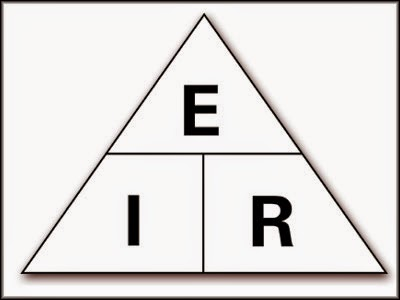constant of proportionality, the resistance, one arrives at the usual mathematical equation that describes this relationship$I = \frac{V}{R},$

where I is the current through the conductor in units of amperes, V is the potential difference measured across the conductor in units of volts, and Ris the resistance of the conductor in units of ohms. More specifically, Ohm's law states that the R in this relation is constant, independent of the current
These units and symbols for electrical quantities will become very important to know as we begin to explore the relationships between them in circuits. The first, and perhaps most important, relationship between current, voltage, and resistance is called Ohm's Law, discovered by Georg Simon Ohm and published in his 1827 paper, The Galvanic Circuit Investigated Mathematically. Ohm's principal discovery was that the amount of electric current through a metal conductor in a circuit is directly proportional to the voltage impressed across it, for any given temperature. Ohm expressed his discovery in the form of a simple equation, describing how voltage, current, and resistance interrelate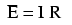In this algebraic expression, voltage (E) is equal to current (I) multiplied by resistance (R). Using algebra techniques, we can manipulate this equation into two variations, solving for I and for R, respectively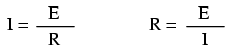Let's see how these equations might work to help us analyze simple circuits

In the above circuit, there is only one source of voltage (the battery, on the left) and only one source of resistance to current (the lamp, on the right). This makes it very easy to apply Ohm's Law. If we know the values of any two of the three quantities (voltage, current, and resistance) in this circuit, we can use Ohm's Law to determine the third
In this first example, we will calculate the amount of current (I) in a circuit, given values of voltage (E) and resistance (R)?What is the amount of current (I) in this circuit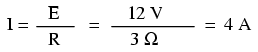In this second example, we will calculate the amount of resistance (R) in a circuit, given values of voltage (E) and current (I)?What is the amount of resistance (R) offered by the lamp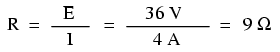In the last example, we will calculate the amount of voltage supplied by a battery, given values of current (I) and resistance (R)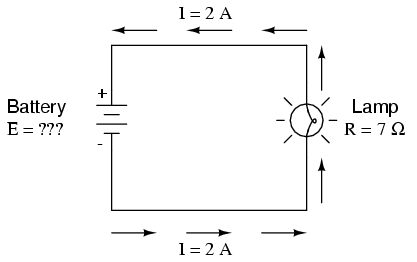?What is the amount of voltage provided by the battery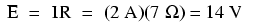Ohm's Law is a very simple and useful tool for analyzing electric circuits. It is used so often in the study of electricity and electronics that it needs to be committed to memory by the serious student. For those who are not yet comfortable with algebra, there's a trick to remembering how to solve for any one quantity, given the other two. First, arrange the letters E, I, and R in a triangle like this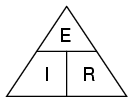If you know E and I, and wish to determine R, just eliminate R from the picture and see what's left

If you know E and R, and wish to determine I, eliminate I and see what's left

Lastly, if you know I and R, and wish to determine E, eliminate E and see what's left